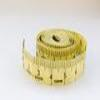#### You may also like### Bigger or Smaller?

When you change the units, do the numbers get bigger or smaller?

### Phenomena to units

Volume of a bacterium - Cubic micrometres $\mathrm{\mu m}^3$
Mass of an oak tree - Tonnes $(10^3\textrm{ kg})$
Area of a leaf - Square centimetres $(\textrm{cm}^2)$
Half-life of Uranium-235 - Millions of years
Area of a football pitch - Hectares
Average life-span of a human -Years
Volume of a bath tub - Cubic metres $(\textrm{m}^3)$

### Units to phenomena

Millimetre - size of an amoeba
Newton - weight of an apple
Angstrom - atomic diameter of a hydrogen atom
Joule - kinetic energy of a table tennis ball travelling at $100\textrm{ km h}^{-1}$
Volt - voltage across an AA cell
Microgram - mass of $1\textrm{ mm}^3$ of air
Micrometre - mitochondrion
Hertz - frequency of tick-tock on a clock
Parsec - distance to Proxima Centauri (closest star other than the Sun)